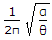# Civil Engineering - Applied Mechanics

### Exercise :: Applied Mechanics - Section 4

21.

According to Kennedy's theorem, if three bodies have plane motions, their instantaneous centres lie on

 A. a point B. a straight line C. two straight lines D. a triangle.

Explanation:

No answer description available for this question. Let us discuss.

22.

A ball of mass 250 g moving on a smooth horizontal table with a velocity of 10 m/sec hits an identical stationary ball B on the table. If the impact is perfectly plastic, the velocity of the ball B just after impact would be

 A. zero B. 5 m/sec C. 10 m/sec D. none of these.

Explanation:

No answer description available for this question. Let us discuss.

23.

If α is the angular acceleration of a compound pendulum whose angular displace ment is θ, the frequency of the motion is

 A. 2πα/θ B.C. 4πα/θ D. 2πα - θ.

Explanation:

No answer description available for this question. Let us discuss.

24.

If two forces each equal to T in magnitude act at right angles, their effect may be neutralised by a third force acting along their bisector in opposite direction whose magnitude will be

 A. 2 T B. 1/2 T C. 2 T D. 3 T E. none of these.

Explanation:

No answer description available for this question. Let us discuss.

25.

Energy may be defined as

 A. power of doing work B. capacity of doing work C. rate of doing work D. all the above.